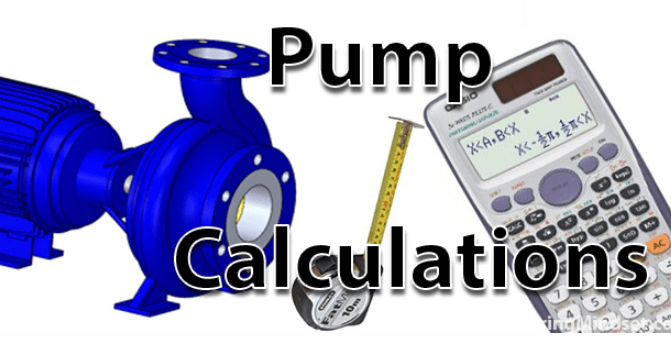# Pump Calculations

Pump Calculations : In this post we will talk about to calculations (new Flow Rate of a Pump from an increase or decrease in Pump speed RPM , new Pump head,new Pump impeller diameter.Pump Calculations

## Pump Calculations

• To calculate the new flow rate of a Pump from an increase or decrease in Pump speed RPM the following formula and calculation can be used.new Pump impeller diameter.

Calculate pump flow rate from an increase or decrease in pump speed RPM

• To calculate the new flow rate of a pump from an increase or decrease in impeller diameter , the  following formula and calculation can be used .
Calculate pump speed RPM with an increase or decrease in flow rate

• To calculate the new pump head pressure from an increase or decrease in pump speed RPM, the following formula and calculation can be used.

Calculate head pressure for an increase or decrease in pump speed rpm

• To calculate the new Pump head pressure from an increase or decrease in flow rate, the following formula and calculation can be used.
Calculate pump head pressure for an increase or decrease in flow rate

• To calculate the new pump head pressure from an increase or decrease in pump speed rpm, the following formula and calculation can be used.

Calculate head pressure for an increase or decrease in pump speed rpm

• To calculate the new pump impeller diameter to suit a change in pump flow ratethe following formula and calculation and be used.
Calculate new pump impeller diameter to suit a change in flow rate